• prewitt算子matlab代码关于计算机视觉的笔记本系列 强调 空间分辨率 图像及其直方图 使用Prewitt运算符检测边缘 使用边缘检测对有缺陷的平板电脑进行分类。 参考： 使用深度神经网络进行分割致谢 我将这项工作献给我...
• prewitt算子matlab代码细胞器状态分析工具 介绍 我们开发了一种拓扑分析工具，该工具能够指示较小的，应力诱导的ER结构变化，而无需涉及荧光强度变化，从而在ER吞噬之前定量识别ER应力。 系统要求 该工具是基于...
• MATLAB中Sobel算子和Prewitt算子的邻域像素点标记如下：edge函数实现的语法格式如下：BW=edge(I, 'sobel')BW=edge(I, 'sobel',thresh)BW=edge (I, 'sobel', thresh,direction)[BW, thresh]=edge (I, 'sobel'…)BW=...

MATLAB中Sobel算子和Prewitt算子的邻域像素点标记如下：
edge函数实现的语法格式如下：
BW=edge(I, 'sobel')
BW=edge(I, 'sobel',thresh)
BW=edge (I, 'sobel', thresh,
direction)
[BW, thresh]=edge (I, 'sobel'…)
BW=edge(I, 'sobel')
自动选择阈值用Sobel算子进行边缘检测。
BW=edge(I,
'sobel',thresh)根据所指定的敏感度阈值thresh，用Sobel算子进行边缘检测，它忽略了所有小于阈值的边缘。当thresh为空时，自动选择阈值。
BW=edge (I, 'sobel', thresh,
direction)根据所指定的敏感度阈值thresh，在所指定的方向direction上，用Sobel
算子进行边缘检测。Direction可取的字符串值为horizontal(水平方向)、vertical(垂直方向)或both(两个方向)。
[BW, thresh]=edge (I, 'sobel'…)返回阈值

展开全文• 图像边缘提取——梯度算子、Roberts算子、prewitt算子、Sobel算子、Kirsch算子、LOG算子的matlab实现
#图像边缘提取—— ##梯度算子、Roberts算子、prewitt算子、Sobel算子、Kirsch算子、LOG算子的matlab实现
matlab代码
clear;
T=20;%阈值
[m,n]=size(f);
%------梯度法-------
f_g=zeros(m,n);
for i=2:m-1
for j=2:n-1
f_g(i,j)=abs(f(i+1,j)-f(i,j))+abs(f(i,j+1)-f(i,j));
if f_g(i,j)<T
f_g(i,j)=0;
else
f_g(i,j)=255;
end
end
end
figure(1);
subplot(2,3,1);imshow(uint8(f_g));title('梯度法');

%------roberts算子-------
f_r=zeros(m,n);
for i=2:m-1
for j=2:n-1
f_r(i,j)=abs(f(i+1,j+1)-f(i,j))+abs(f(i,j+1)-f(i+1,j));
if f_r(i,j)<T
f_r(i,j)=0;
else
f_r(i,j)=255;
end
end
end
%f_r=imbinarize(imfilter(f,r),T);
subplot(2,3,2);imshow(uint8(f_r));title('Roberts算子');

%------prewitt算子-------
f_p=zeros(m,n);
for i=2:m-1
for j=2:n-1
f_p(i,j)=abs(f(i-1,j-1)+f(i,j-1)+f(i+1,j-1)-f(i-1,j+1)-f(i,j+1)-f(i+1,j+1))+abs(f(i+1,j-1)+f(i+1,j)+f(i+1,j+1)-f(i-1,j-1)-f(i-1,j)-f(i-1,j+1));
if f_p(i,j)<15
f_p(i,j)=0;
else
f_p(i,j)=255;
end
end
end
subplot(2,3,3);imshow(uint8(f_p));title('Prewitt算子');

%------sobel算子-------
f_s=zeros(m,n);
for i=2:m-1
for j=2:n-1
f_s(i,j)=abs(f(i-1,j-1)+2*f(i,j-1)+f(i+1,j-1)-f(i-1,j+1)-2*f(i,j+1)-f(i+1,j+1))+abs(f(i+1,j-1)+2*f(i+1,j)+f(i+1,j+1)-f(i-1,j-1)-2*f(i-1,j)-f(i-1,j+1));
if f_s(i,j)<T
f_s(i,j)=0;
else
f_s(i,j)=255;
end
end
end
subplot(2,3,4);imshow(uint8(f_s));title('Sobel算子');

%------krisch算子-------
k(:,:,1)=[-3,-3,-3;
-3,0,5;
-3,5,5];
k(:,:,2)=[-3,-3,5;
-3,0,5;
-3,-3,5];
k(:,:,3)=[-3,5,5;
-3,0,5;
-3,-3,-3];
k(:,:,4)=[-3,-3,-3;
-3,0,-3;
5,5,5];
k(:,:,5)=[5,5,5;
-3,0,-3;
-3,-3,-3];
k(:,:,6)=[-3,-3,-3;
5,0,-3;
5,5,-3];
k(:,:,7)=[5,-3,-3;
5,0,-3;
5,-3,-3];
k(:,:,8)=[5,5,-3;
5,0,-3;
-3,-3,-3];
kk=zeros(size(f));
I=double(f);
for i=1:8
f_k(:,:,i)=conv2(I,k(:,:,i),'same');
kk=max(kk,f_k(:,:,i));
end
f_kk=imbinarize(kk,600);
subplot(2,3,5);imshow(f_kk);title('Krisch算子');

%------LoG算子-------
log1=[0 0 -1 0 0;
0 -1 -2 -1 0;
-1 -2 16 -2 -1;
0 -1 -2 -1 0;
0 0 -1 0 0];

f_l=conv2(f,log1,'same');
f_ll=imbinarize(abs(f_l),300);
subplot(2,3,6);imshow(f_ll);title('LoG算子');


效果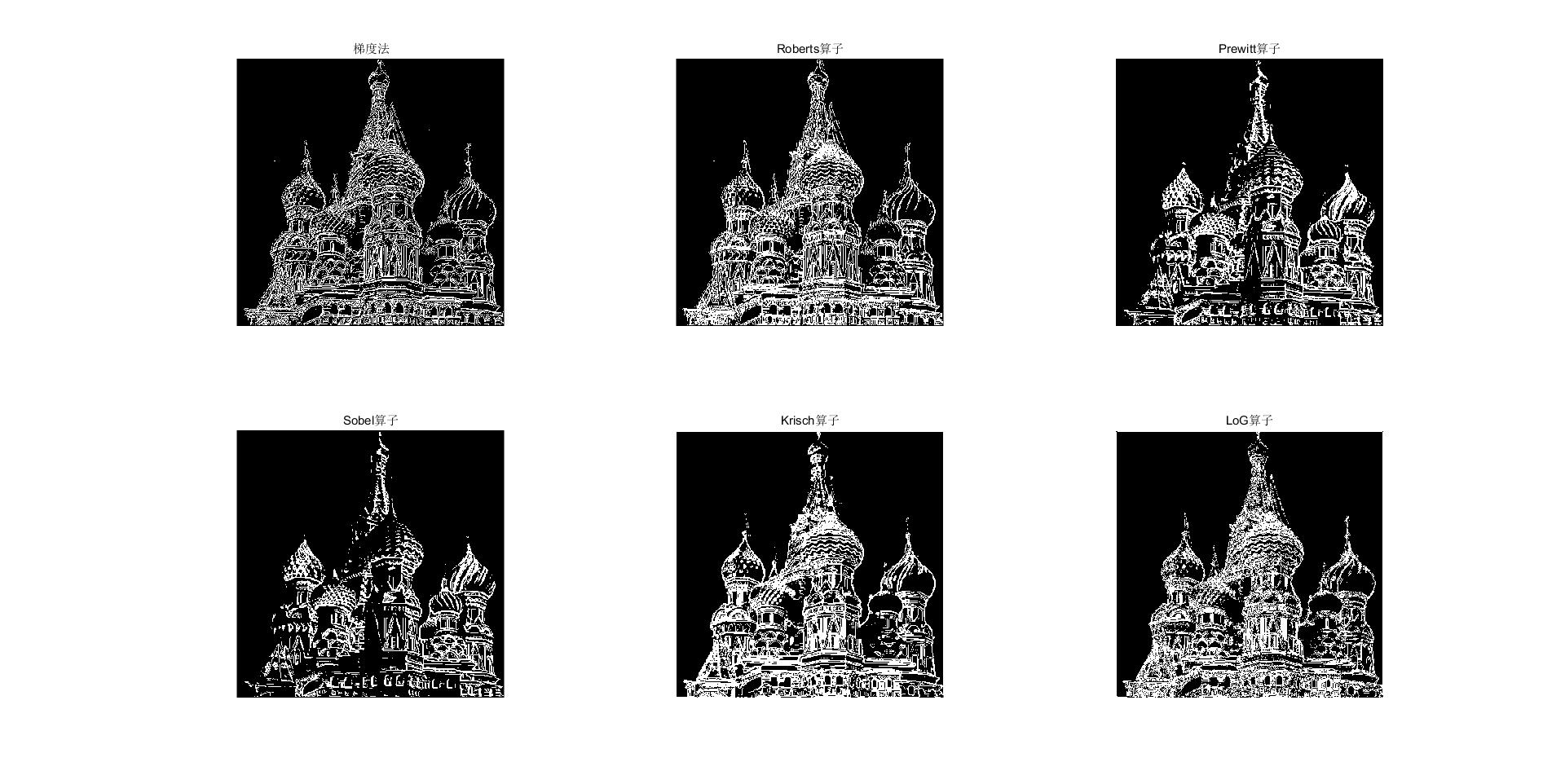展开全文数字图像处理 边缘检测算法
• 自编matlab下的边缘检测算法，以prewitt算子示例
• 本次代码共包含5个程序，均由MATLAB实现，其功能为图像锐化。包含laplacian算子、Prewitt算子、Robert算子、sobel算子实现图像锐化，并有一维和二维laplacian算子对比。内还有各个算子的运算过程（Word文档）
• Matlab采用梯度算子、拉普拉斯算子、Sobel算子及Prewitt算子对图像进行锐化 文章目录Matlab采用梯度算子、拉普拉斯算子、Sobel算子及Prewitt算子对图像进行锐化梯度算子拉普拉斯算子Robel算子Prewitt算子整体程序：...
Matlab采用梯度算子、拉普拉斯算子、Sobel算子及Prewitt算子对图像进行锐化

文章目录
Matlab采用梯度算子、拉普拉斯算子、Sobel算子及Prewitt算子对图像进行锐化梯度算子拉普拉斯算子Robel算子Prewitt算子整体程序：结果显示：

梯度算子
设图像为f(x,y), 定义为f(x,y)在点(x,y)的梯度矢量为G[f(x,y)]：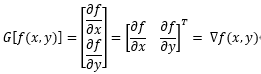性质： 1）梯度的方向是在f(x,y)的最大变化率方向上 2）梯度的幅度用G[f(x,y)]表示：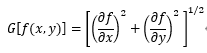梯度 对于数字图像：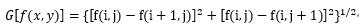简化为：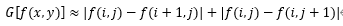Roberts梯度（交叉梯度） 对于数字图像：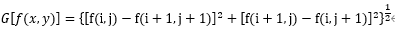简化为：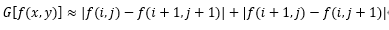拉普拉斯算子
拉普拉斯算子：
线性、各向同性（旋转不变）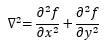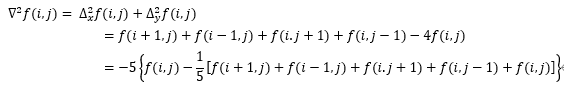对于数字图像f(i,j)，其一阶导数：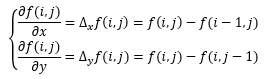二阶偏导数为：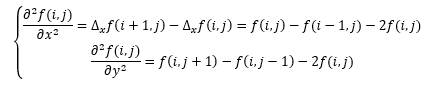拉普拉斯算子模板表示：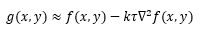注意： 1）有时g(x,y)输出小或为负 2）有时g(x,y)的增强可能超出范围，需要变回0~L-1尺度中
Robel算子
基本思想： 以待增强图像的任意像素(i,j)为中心，截取一个3X3的像素窗口，先分别计算窗口中像素在x，y方向的梯度：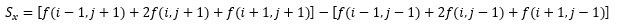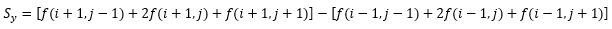增强后(i,j)点的亮度：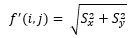可简化为：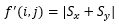优点： 1）由于引入了加权平均，所以对图像中的随机噪声具有一定的平滑作用 2）由于采用间隔两行或两列的查分，边缘两侧的像素得到增强，锐化图像的边缘显得粗而亮 S_x，S_y可用卷积模板来实现：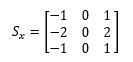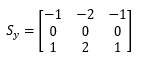可见：其重点放在接近于模板中心的像素点
Prewitt算子
基本思想： 与Sobel算子相同，方程的形式相同，但其中系数不同：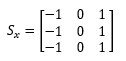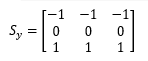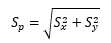可见：与Sobel算子不同，其重点没有放在接近于模板中心的像素点
整体程序：
clc;
clear all;
img = rgb2gray(img);
subplot(2,3,1);
imshow(img);
title('原始图像');
[m,n] = size(img);
T = 20;

%Roberts算子
imgr = zeros(m,n);
for i=2:m-1
for j=2:n-1
imgr(i,j)= abs(img(i,j)-img(i+1,j+1)) + abs(img(i+1,j)-img(i,j+1));
if imgr(i,j)<T
imgr(i,j) = 0;
else
imgr(i,j) = 255;
end
end
end
subplot(2,3,2);
imshow(imgr);
title('Roberts算子图像');

%Laplace算子
imgl = zeros(m,n);
for i=2:m-1
for j=2:n-1
imgl(i,j)= abs(img(i+1,j)+img(i-1,j)+img(i,j+1)+img(i,j-1)-4*img(i,j));
if imgl(i,j)<T
imgl(i,j) = 0;
else
imgl(i,j) = 255;
end
end
end
subplot(2,3,3);
imshow(imgl);
title('Laplace算子图像');

%Sobel算子
imgs = zeros(m,n);
for i=2:m-1
for j=2:n-1
imgs(i,j)= abs(img(i-1,j+1)+2*img(i,j+1)+img(i+1,j+1)-img(i-1,j-1)-2*img(i,j-1)-img(i+1,j-1)) + abs(img(i+1,j-1)+2*img(i+1,j)+img(i+1,j+1)-img(i-1,j-1)-2*img(i-1,j)-img(i-1,j+1));
if imgs(i,j)<T
imgs(i,j) = 0;
else
imgs(i,j) = 255;
end
end
end
subplot(2,3,4);
imshow(imgs);
title('Sobel算子图像');

%Prewitt算子
imgp = zeros(m,n);
for i=2:m-1
for j=2:n-1
imgp(i,j)= abs(img(i-1,j+1)+img(i,j+1)+img(i+1,j+1)-img(i-1,j-1)-img(i,j-1)-img(i+1,j-1)) + abs(img(i+1,j-1)+img(i+1,j)+img(i+1,j+1)-img(i-1,j-1)-img(i-1,j)-img(i-1,j+1));
if imgp(i,j)<T
imgp(i,j) = 0;
else
imgp(i,j) = 255;
end
end
end
subplot(2,3,5);
imshow(imgp);
title('Prewitt算子图像');

结果显示：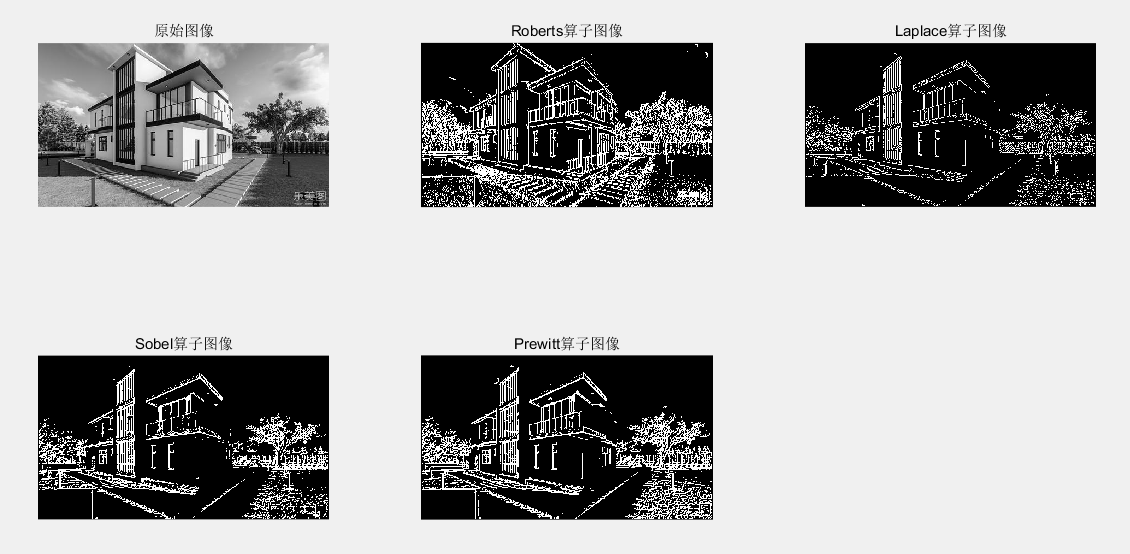展开全文• 由于Prewitt算子采用 3x3 模板对区域内的像素值进行计算，而Robert算子的模板为 2x2，故Prewitt算子的边缘检测结果在水平方向和垂直方向均比Robert算子更加明显。Prewitt算子适合用来识别噪声较多、灰度渐变的图像，...
Prewitt 算子
1. 原理
Prewitt算子是一种图像边缘检测的微分算子，其原理是利用特定区域内像素灰度值产生的差分实现边缘检测。由于Prewitt算子采用 3x3 模板对区域内的像素值进行计算，而Robert算子的模板为 2x2，故Prewitt算子的边缘检测结果在水平方向和垂直方向均比Robert算子更加明显。Prewitt算子适合用来识别噪声较多、灰度渐变的图像，其计算公式如下所示：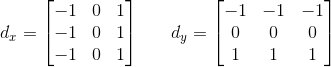例如，下面给出Prewitt算子的模板，在像素点P5处 x 和 y 方向上的梯度大小 g_x 和 g_y 分别计算为：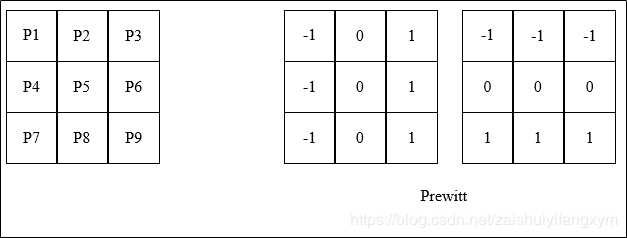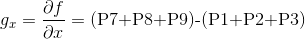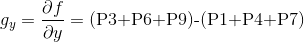2. pytohn代码
# -*- coding: utf-8 -*-
import cv2
import numpy as np
import matplotlib.pyplot as plt

# 读取图像
img_RGB = cv2.cvtColor(img, cv2.COLOR_BGR2RGB)

# 灰度化处理图像
grayImage = cv2.cvtColor(img, cv2.COLOR_BGR2GRAY)

# Prewitt算子
kernelx = np.array([[1, 1, 1], [0, 0, 0], [-1, -1, -1]], dtype=int)
kernely = np.array([[-1, 0, 1], [-1, 0, 1], [-1, 0, 1]], dtype=int)
x = cv2.filter2D(grayImage, cv2.CV_16S, kernelx)
y = cv2.filter2D(grayImage, cv2.CV_16S, kernely)
# 转uint8
absX = cv2.convertScaleAbs(x)
absY = cv2.convertScaleAbs(y)
Prewitt = cv2.addWeighted(absX, 0.5, absY, 0.5, 0)

# 用来正常显示中文标签
plt.rcParams['font.sans-serif'] = ['SimHei']

# 显示图形
plt.subplot(121),plt.imshow(img_RGB),plt.title('原始图像'), plt.axis('off') #坐标轴关闭
plt.subplot(122),plt.imshow(Prewitt, cmap=plt.cm.gray ),plt.title('Prewitt算子'), plt.axis('off')
plt.show()

效果图：3. matlab代码
I =imread('C:/Users/123/Pictures/66.png');
I0=rgb2gray(I);
BW1 =edge(I0,'prewitt');
subplot(121),imshow(I0);title('原始图像');
subplot(122),imshow(BW1);title('prewitt算子');

效果图：展开全文• 原理使用prewitt算子找到图像边缘。设定阈值，并根据阈值将边缘转化成二值数组。将二值数组中值为1的像素以原灰度值显示，值为0的像素灰度值置0显示。注：因为选择的是白纸黑字的图像，所以边缘实际上就是图像前景，...
• Matlab数字图像处理——Sobel算子锐化、Prewitt算子锐化
• 经典的图像边缘检测算子，值得参考。很有借鉴意义。matlab函数形式
• 请用MATLAB或其它语言编写相关程序，输入一幅灰度图像，分别采用梯度算子、拉普拉斯算子、Sobel算子、Prewitt算子对图像进行锐化，在同一个窗口输出显示原始图像和４种结果图像。
• ## Prewitt算子的运用

千次阅读 2015-07-15 14:46:57
Prewitt算子折叠 是一种一阶微分算子的边缘检测，利用像素点上下、左右邻点的灰度差，在边缘处达到 极值检测边缘，去掉部分伪边缘，对噪声具有平滑作用 。其原理是在图像空间利用两个方向模板与图 像...
• Robert算子、sobel算子、Prewitt算子、canny边缘检测算子 之前做的笔记，懒得再敲一遍了，先传上来，方便自己以后加深记忆图像处理
• Prewitt算子 7. 拉普拉斯算子 8. matlab代码实现 1. 锐化 1.锐化(Sharpening) ：图像在传输或变换过程中（如未聚焦好）、受到各种干扰而退化，典型的是图像模糊，而图像的判读和识别中，常需突出目标的轮廓或边缘...
• 学习DIP第47天 转载请标明本文出处：http://blog.csdn.net/tonyshengtan ，出于尊重文章作者的劳动，转载请标明出处！文章代码已托管，欢迎共同开发：... ... ...依然是废话，这篇主要想对比下Sobel，Prewitt和Scharr...图像处理 Sobel算子 Scharr算子
• MATLAB 函数 将图像 I 转换为双精度。I 可以是灰度强度图像、真彩色图像或二值图像。 im2double 将整数数据类型的输出重新缩放到范围 [0, 1]。 调用格式如下： I2 = im2double(I) I2 = im2double(I,'indexed') I...计算机视觉 深度学习 机器视觉 图像处理
• 6. Prewitt算子的实现 使用分别使用Prewitt1算子、Prewitt2算子作为模板，用来对图像进行边缘检测，水平+竖直边缘 # prewitt 算子 prewitt_1 = np.array( [[-1, 0, 1], [-1, 0, 1], [-1, 0, 1]] ) ...
• Prewitt算子 为了提高精度，对于每个像素考虑它与周围8个邻域中像素值的加权差，卷积核Gx=[1,0,-1;1,0,-1;1,0,-1];Gy=[-1,-1,-1;0,0,0;1,1,1] Sobel算子 Sobel算子与Prewitt算子相同，考虑周围8个像素的加权差，...
• 经典边缘检测算子的实现Sobel prewitt，canny，roberts边缘检测
• 不用matlab的自带函数，自编的边缘提取程序。 不用matlab的自带函数，自编的边缘提取程序。 不用matlab的自带函数，自编的边缘提取程序。 不用matlab的自带函数，自编的边缘提取程序。
• 相比Roberts算子，Prewitt算子对噪声有抑制作用，抑制噪声的原理是通过像素平均，因此噪声较多的图像处理得比较好，但是像素平均相当于对图像的低通滤波，所以Prewitt算子对边缘的定位却不如Roberts算子。...
• 边缘检测是图像处理和计算机视觉中的基本问题，边缘检测的目的...Roberts Cross算子，Prewitt算子，Sobel算子， Kirsch算子，罗盘算子；二阶： Marr-Hildreth，在梯度方向的二阶导数过零点，Canny算子，Laplacian算子。
• title('Prewitt算子边缘检测');   % BW4=edge(I0,'LOG',threshold); % subplot(235); % imshow(BW4); % title('LOG算子边缘检测')   BW5=edge(I0,'Canny',threshold); subplot(224); imshow(BW5...
• 用三个算子。 1 sobel算子提取结果。 2 roberts算子提取结果 3 prewitt算子提取结果
• matlab编写的Roberts,prewitt,Sobel,Laplacian算子程序，主要用于图像锐化代码，有效果图。...

# prewitt算子matlabmatlab 订阅